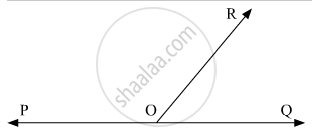# Given ∠Por = 3x And ∠Qor = 2x + 10°. If Poq Is a Straight Line, Then the Value Of X Is - Mathematics

MCQ

Given ∠POR = 3x and ∠QOR = 2x + 10°. If POQ is a straight line, then the value of x is

#### Options

• 30°

• 34°

• 36°

• none of these

#### Solution

Let us draw the following figure, showing POQ as a straight line.Thus, ∠POR and ∠QORform a linear pair, therefore their sum must be supplementary. That is;

∠POR + ∠QOR = 180°

It is given that

∠POR = 3x

and ∠QOR = 2x + 10°

On substituting these two values above, we get:

3x +2x + 10° = 180°

5x +10° = 180°

5x = 180° -10°

5x = 170°

x = (170°)/5

x = 34°

Concept: Concept of Parallel Lines
Is there an error in this question or solution?

#### APPEARS IN

RD Sharma Mathematics for Class 9
Chapter 10 Lines and Angles
Q 5 | Page 51